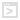## Definition

Adds indicator data to the strategy's machine learning model. This is needed both in creating a new model and using an existing model to predict long or short signals.

 Note:  The AddDSTIndicator method has two overrides. The min and max parameters are optional and are used when normalizing the data before creating a new machine learning model. Normalizing the data is the process of setting all of the data values to values between 0 and 1 before feeding the data set to the machine learning model. By default these values will be set to 0 for the minimum and double the maximum value found in the training data set. The min and max can be modified if you feel that the maximum value may be more than double the maximum value from the training set. As an example, if you trained the model based on the Close value for an instrument from 2000-2005 the maximum for the Close was \$118.00 then we will use \$236.00 for the default maximum. If we are running the strategy live we still normalize the data before sending the values to the model. If the current Close is 300, then when we normalize the data, the normalized value will always be 1 since it is more than the expected maximum of \$236 and the model may not perform as expected.

## Syntax

AddDSTIndicator(ISeries<double> dataSeries, string name, int decimalPrecision)
AddDSTIndicator(ISeries<double> dataSeries, string name, int decimalPrecision, double min, double max)

 Warning: This method should ONLY be called within the OnStateChange() method during State.Configure

## Parameters

 dataSeries An ISeries data series used to pass indicator data to the machine learning model. The value must be a data series such as Volume or MACD(1, 26, 9) and not the current bar's value such as Volume or MACD(1, 26, 0). name A string value representing the name of the indicator data series such as "Volume". Please use the same name when creating a model as when you predict a model. When selecting a Prediction strategy, the Deep Signal Library will look for strategies with the same number of parameter and the same name to find matching create/predict strategies when populating the Strategy Data Folder in Settings. decimalPrecision An int value representing the number of decimal places of precision for the series. For Volume this might be 0, meaning the data will be rounded to whole numbers. As an example for decimalPrecision equal to 2, the values from the series will be rounded to 2 decimal places. min A double value representing the minimum value for the series found before training the machine learning model. By default this value is set to 0 when creating the machine learning model.
 max A double value representing the maximum value for the series when training the machine learning model. By default this value is set to double the value found in the data set before training the model. As an example, if we used Volume for the indicator data and used the time period of 1/1/18 through 1/1/20 for the training data. If the highest volume we found for this time period was 1,000,000 shares then we'll use 2,000,000 for the default maximum value.

##Adding indicator data to a machine learning model

protected override void OnStateChange()
{

// Please do not change, the DST Library will need to call OnStateChange

base.OnStateChange();

if (State == State.Configure)
{
// AddDSTIndicator adds any series value that you want to use in training a machine learning model

AddDSTIndicator(MACD(1, 26, 9).Diff, "MACD Diff", 4);
}
}

##Adding indicator data to machine learning model with min and max parameters

protected override void OnStateChange()
{
// Please do not change, the DST Library will need to call OnStateChange

base.OnStateChange();

if (State == State.Configure)
{
//  AddDSTIndicator adds any series value that you want to use in training a machine learning model

//  The example below will set the number of decimal place precision to 0 which will round all values

//  to whole numbers. The min value will be set to 0 and max value will be set to 3,000,000

}
}

Futures, foreign currency and options trading contains substantial risk and is not for every investor. An investor could potentially lose all or more than the initial investment. Risk capital is money that can be lost without jeopardizing ones financial security or lifestyle. Only risk capital should be used for trading and only those with sufficient risk capital should consider trading. Past performance is not necessarily indicative of future results.